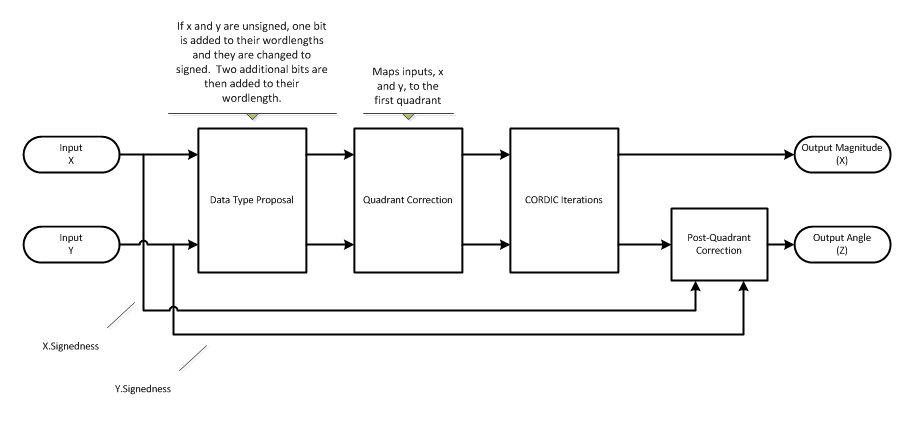# cordicabs

CORDIC-based absolute value

## Syntax

``r = cordicabs(c)``
``r = cordicabs(c,niters)``
``r = cordicabs(___,'ScaleOutput',b)``

## Description

example

````r = cordicabs(c)` returns the magnitude of the complex elements of `c`.```
````r = cordicabs(c,niters)` performs the number of CORDIC algorithm iterations specified by `niters`.```
````r = cordicabs(___,'ScaleOutput',b)` specifies whether to scale the output by the inverse CORDIC gain factor.```

## Examples

collapse all

Compare the absolute value computed using double-precision input values to the `cordicabs` and `abs` functions.

`dblValues = complex(rand(5,4),rand(5,4))`
```dblValues = 5×4 complex 0.8147 + 0.6557i 0.0975 + 0.7577i 0.1576 + 0.7060i 0.1419 + 0.8235i 0.9058 + 0.0357i 0.2785 + 0.7431i 0.9706 + 0.0318i 0.4218 + 0.6948i 0.1270 + 0.8491i 0.5469 + 0.3922i 0.9572 + 0.2769i 0.9157 + 0.3171i 0.9134 + 0.9340i 0.9575 + 0.6555i 0.4854 + 0.0462i 0.7922 + 0.9502i 0.6324 + 0.6787i 0.9649 + 0.1712i 0.8003 + 0.0971i 0.9595 + 0.0344i ```
`r_dbl_ref = abs(dblValues)`
```r_dbl_ref = 5×4 1.0458 0.7640 0.7234 0.8356 0.9065 0.7936 0.9711 0.8128 0.8586 0.6730 0.9964 0.9691 1.3064 1.1604 0.4876 1.2371 0.9277 0.9800 0.8062 0.9601 ```
`r_dbl_cdc = cordicabs(dblValues)`
```r_dbl_cdc = 5×4 1.0458 0.7640 0.7234 0.8356 0.9065 0.7936 0.9711 0.8128 0.8586 0.6730 0.9964 0.9691 1.3064 1.1604 0.4876 1.2371 0.9277 0.9800 0.8062 0.9601 ```

Compute absolute values of fixed-point inputs.

`fxpValues = fi(dblValues)`
```fxpValues = 0.8147 + 0.6557i 0.0975 + 0.7578i 0.1576 + 0.7061i 0.1419 + 0.8235i 0.9058 + 0.0357i 0.2785 + 0.7431i 0.9706 + 0.0318i 0.4218 + 0.6948i 0.1270 + 0.8491i 0.5469 + 0.3922i 0.9572 + 0.2769i 0.9157 + 0.3171i 0.9134 + 0.9340i 0.9575 + 0.6555i 0.4854 + 0.0462i 0.7922 + 0.9502i 0.6324 + 0.6787i 0.9649 + 0.1712i 0.8003 + 0.0971i 0.9595 + 0.0345i DataTypeMode: Fixed-point: binary point scaling Signedness: Signed WordLength: 16 FractionLength: 15 ```
`r_fxp_cdc = cordicabs(fxpValues)`
```r_fxp_cdc = 1.0458 0.7640 0.7234 0.8356 0.9066 0.7935 0.9712 0.8128 0.8586 0.6730 0.9965 0.9691 1.3064 1.1604 0.4876 1.2371 0.9277 0.9799 0.8062 0.9601 DataTypeMode: Fixed-point: binary point scaling Signedness: Signed WordLength: 18 FractionLength: 15 ```

## Input Arguments

collapse all

Complex input array, specified as a scalar, vector, matrix, or multidimensional array. All input values must have the same data type.

Data Types: `single` | `double` | `int8` | `int16` | `int32` | `int64` | `uint8` | `uint16` | `uint32` | `uint64` | `fi`
Complex Number Support: Yes

Number of iterations the CORDIC algorithm performs, specified as a positive integer-valued scalar.

If you do not specify `niters`, or if you specify a value that is too large, the algorithm uses a maximum value. For fixed-point operation, the maximum number of iterations is one less than the word length of `c`. For floating-point operation, the maximum value is `52` for double or `23` for single.

Increasing the number of iterations can produce more accurate results but also increases the expense of the computation and adds latency.

Whether to scale output by inverse CORDIC gain factor, specified as a numeric or logical `1` (`true`) or `0` (`false`).

Data Types: `logical`

## Output Arguments

collapse all

Magnitude values of the complex input values, returned as a scalar, vector, matrix, or multidimensional array. If the input `c` is fixed point, then `r` is returned as a signed fixed-point data type using binary-point scaling. If the inputs are signed, then the word length of `r` is the input word length + 2. If the inputs are unsigned, then the word length of `r` is the input word length + 3. The fraction length of `r` is always the same as the fraction length of the input `c`.

collapse all

### CORDIC

CORDIC is an acronym for COordinate Rotation DIgital Computer. The Givens rotation-based CORDIC algorithm is one of the most hardware-efficient algorithms available because it requires only iterative shift-add operations (see References). The CORDIC algorithm eliminates the need for explicit multipliers. Using CORDIC, you can calculate various functions such as sine, cosine, arcsine, arccosine, arctangent, and vector magnitude. You can also use this algorithm for divide, square root, hyperbolic, and logarithmic functions.

Increasing the number of CORDIC iterations can produce more accurate results but also increases the expense of the computation and adds latency.

## Algorithms

collapse all

### Signal Flow DiagramsCORDIC Vectoring KernelThe accuracy of the CORDIC kernel depends on the choice of initial values for X, Y, and Z. This algorithm uses the following initial values:

### fimath Propagation Rules

CORDIC functions discard any local `fimath` attached to the input.

The CORDIC functions use their own internal `fimath` when performing calculations:

• `OverflowAction``Wrap`

• `RoundingMethod``Floor`

The output has no attached `fimath`.

 Volder, Jack E. “The CORDIC Trigonometric Computing Technique.” IRE Transactions on Electronic Computers. EC-8, no. 3 (Sept. 1959): 330–334.

 Andraka, Ray. “A Survey of CORDIC Algorithm for FPGA Based Computers.” In Proceedings of the 1998 ACM/SIGDA Sixth International Symposium on Field Programmable Gate Arrays, 191-200. https://dl.acm.org/doi/10.1145/275107.275139.

 Walther, J.S. “A Unified Algorithm for Elementary Functions.” In Proceedings of the May 18-20, 1971 Spring Joint Computer Conference, 379-386. https://dl.acm.org/doi/10.1145/1478786.1478840.

 Schelin, Charles W. “Calculator Function Approximation.” The American Mathematical Monthly, no. 5 (May 1983): 317-325. https://doi.org/10.2307/2975781.